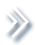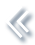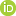####ArchivesReview Articles

## Multivariate extremes and max-stable processes: discussion of the paper by Zhengjun Zhang

R. L. Smith

Department of Statistics and Operations Research, University of North Carolina, Chapel Hill, NC 27514, USAPages 41-44 | Received 01 Jan. 2021, Accepted 01 Jan. 2021, Published online: 20 Jan. 2021,
• Abstract
• References
• Citations

Abstract

This discussion reviews the paper by Zhengjun Zhang in the context of broader research on multivariate extreme value theory and max-stable processes.

## References

1. Blanchet, J., & Creutin, J.-D. (2017). Co-occurrence of extreme daily rainfall in the French Mediterranean region. Water Resources Research53(11), 9330–9349. https://doi.org/10.1002/2017WR020717 [Crossref][Web of Science ®], [Google Scholar]
2. Blanchet, J., & Davison, A. C. (2011). Spatial modeling of extreme snow depth. The Annals of Applied Statistics5(3), 1699–1725. https://doi.org/10.1214/11-AOAS464 [Crossref][Web of Science ®], [Google Scholar]
3. Bopp, G., Shaby, B., & Huser, R. (2018). A hierarchical max-infinitely divisible process for extreme areal precipitation over watersheds, Arxiv:1804.04588. [Google Scholar]
4. Brown, B. M., & Resnick, S. (1977). Extreme values of independent stochastic processes. Journal of Applied Probability14(4), 732–739. https://doi.org/10.2307/3213346 [Crossref][Web of Science ®], [Google Scholar]
5. Coles, S. G., & Tawn, J. A. (1991). Modelling extreme multivariate events. Journal of the Royal Statistical Society, Series B53(2), 377–392. https://doi.org/10.1111/j.2517-6161.1991.tb01830.x [Google Scholar]
6. Cooley, D., Cisewski, J., Erhardt, R. J., Jeon, S., Mannshardt, E., Omolo, B. O., & Sun, Y. (2012). A survey of spatial extremes: Measuring spatial dependence and modeling spatial effects. REVSTAT10(1), 135–165. [Web of Science ®], [Google Scholar]
7. Davison, A. C., Huser, R., & Thibaud, E. (2019). Spatial extremes. In A. Gelfand, M. Fuentes, J. Hoeting, & R. Smith (Eds.), Handbook of environmental and ecological statistics (pp. 711–744). [Crossref], [Google Scholar]
8. Davison, A. C., Padoan, S. A., & Ribatet, M. (2012). Statistical modeling of spatial extremes. Statistical Science27(2), 161–186. https://doi.org/10.1214/11-STS376 [Crossref][Web of Science ®], [Google Scholar]
9. Davison, A. C., & Smith, R. L. (1990). Models for exceedances over high thresholds (with discussion). Journal of the Royal Statistical Society, Series B52(3), 393–442. https://doi.org/10.1111/j.2517-6161.1990.tb01796.x [Google Scholar]
10. de Haan, L., & Pickands, J. (1986). Stationary min-stable stochastic processes. Probability Theory and Related Fields72(4), 477–492. https://doi.org/10.1007/BF00344716 [Crossref][Web of Science ®], [Google Scholar]
11. Deheuvels, P. (1978). Caracterisation complète des lois extrêmes multivariées et de la convergence des types extrêmes. Publications de l'Institut de statistique de l'Université de Paris23, 1–36. [Google Scholar]
12. Deheuvels, P. (1983). Point processes and multivariate extreme values. Journal of Multivariate Analysis13(2), 257–272. https://doi.org/10.1016/0047-259X(83)90025-8 [Crossref][Web of Science ®], [Google Scholar]
13. Fisher, R. A., & Tippett, L. H. C. (1928). Limiting forms of the frequency distribution of the largest or smallest member of a sample. Mathematical Proceedings of the Cambridge Philosophical Society24(2), 180–190. https://doi.org/10.1017/S0305004100015681 [Crossref], [Google Scholar]
14. Giné, E., Hahn, M. G., & Vatan, P. (1990). Max-infinitely divisible and max-stable sample continuous processes. Probability Theory and Related Fields87(2), 139–165. https://doi.org/10.1007/BF01198427 [Crossref][Web of Science ®], [Google Scholar]
15. Gnedenko, B. (1943). Sur la distribution limite du terme maximum d'une série aléatoire. The Annals of Mathematics44(3), 423–453. https://doi.org/10.2307/1968974 [Crossref], [Google Scholar]
16. Haan, L. D. (1984). A spectral representation for max-stable processes. The Annals of Probability12(4), 1194–1204. https://doi.org/10.1214/aop/1176993148 [Crossref][Web of Science ®], [Google Scholar]
17. Haan, L., & Resnick, S. I. (1977). Limit theory for multivariate sample extremes. Zeitschrift für Wahrscheinlichkeitstheorie und Verwandte Gebiete40(4), 317–337. https://doi.org/10.1007/BF00533086 [Crossref], [Google Scholar]
18. Heffernan, J., Tawn, J. A., & Zhang, Z. (2007). Asymptotically (in)dependent multivariate maxima of moving maxima processes. Extremes10(1–2), 57–82. https://doi.org/10.1007/s10687-007-0035-1 [Crossref], [Google Scholar]
19. Huser, R., & Davison, A. C. (2013). Composite likelihood estimation for the Brown-Resnick process. Biometrika100, 511–518. https://doi.org/10.1093/biomet/ass089 [Crossref][Web of Science ®], [Google Scholar]
20. Huser, R., & Davison, A. C. (2014). Space-time modelling of extreme events. Journal of the Royal Statistical Society: Series B (Statistical Methodology)76(2), 439–461. https://doi.org/10.1111/rssb.2014.76.issue-2 [Crossref][Web of Science ®], [Google Scholar]
21. Huser, R., Opitz, T., & Thibaud, E. (2020). Max-infinitely divisible models and inference for spatial extremes. Scandinavian Journal of Statistics[Crossref][Web of Science ®], [Google Scholar]
22. Joe, H., Smith, R. L., & Weissman, I. (1992). Bivariate threshold methods for extremes. Journal of the Royal Statistical Society, Series B54(1), 171–183. https://doi.org/10.1111/j.2517-6161.1992.tb01871.x [Google Scholar]
23. Kabluchko, Z., Schlather, M., & De Haan, L. (2009). Stationary max-stable fields associated to negative definite functions. The Annals of Probability37(5), 2042–2065. https://doi.org/10.1214/09-AOP455 [Crossref][Web of Science ®], [Google Scholar]
24. Ledford, A. W., & Tawn, J. A. (1996). Statistics for near independence in multivariate extreme values. Biometrika83(1), 169–187. https://doi.org/10.1093/biomet/83.1.169 [Crossref][Web of Science ®], [Google Scholar]
25. Ledford, A. W., & Tawn, J. A. (1997). Modelling dependence within joint tail regions. Journal of the Royal Statistical Society: Series B (Statistical Methodology)59(2), 475–499. https://doi.org/10.1111/rssb.1997.59.issue-2 [Crossref], [Google Scholar]
26. Ledford, A. W., & Tawn, J. A. (2003). Diagnostics for dependence within time series extremes. Journal of the Royal Statistical Society: Series B (Statistical Methodology)65(2), 521–543. https://doi.org/10.1111/rssb.2003.65.issue-2 [Crossref][Web of Science ®], [Google Scholar]
27. Oesting, M., Schlather, M., & Friedrichs, P. (2017). Statistical post-processing of forecasts for extremes using bivariate Brown-Resnick processes with an application to wind gusts. Extremes20, 309–332. https://doi.org/10.1007/s10687-016-0277-x [Crossref][Web of Science ®], [Google Scholar]
28. Opitz, T. (2013). Extremal t processes: Elliptical domain of attraction and a spectral representation. Journal of Multivariate Analysis122, 409–413. https://doi.org/10.1016/j.jmva.2013.08.008 [Crossref][Web of Science ®], [Google Scholar]
29. Padoan, S. A., Ribatet, M., & Sisson, S. A. (2010). Likelihood-based inference for max-stable processes. Journal of the American Statistical Association105(489), 263–277. https://doi.org/10.1198/jasa.2009.tm08577 [Taylor & Francis Online][Web of Science ®], [Google Scholar]
30. Penrose, M. D. (1992). Semi-min-stable processes. The Annals of Probability20(3), 1450–1463. https://doi.org/10.1214/aop/1176989700 [Crossref][Web of Science ®], [Google Scholar]
31. Pickands, J. (1981). Multivariate extreme value distributions. Bulletin of the International Statistical Institute49, 859–878. [Google Scholar]
32. Pickands, J. (1989). Multivariate negative exponential and extreme value distributions. In J. Hüsler and R. Reiss (Eds.), Extreme value theory: Lecture notes in statistics (p. 51). Springer. [Google Scholar]
33. Pickands, J. P., III. (1975). Statistical inference using extreme order statistics. The Annals of Statistics3(1), 119–131. https://doi.org/10.1214/aos/1176343003 [Crossref][Web of Science ®], [Google Scholar]
34. Prescott, P., & Walden, A. T. (1980). Maximum likelihood estimation of the parameters of the generalized extreme-value distribution. Biometrika67(3), 723–724. https://doi.org/10.1093/biomet/67.3.723 [Crossref][Web of Science ®], [Google Scholar]
35. Prescott, P., & Walden, A.T. (1983). Maximum likeiihood estimation of the parameters of the three-parameter generalized extreme-value distribution from censored samples. Journal of Statistical Computation and Simulation16(3–4), 241–250. https://doi.org/10.1080/00949658308810625 [Taylor & Francis Online][Web of Science ®], [Google Scholar]
36. Reich, B. J., & Shaby, B. A. (2019). A spatial Markov model for climate extremes. Journal of Computational and Graphical Statistics28(1), 117–126. https://doi.org/10.1080/10618600.2018.1482764 [Taylor & Francis Online][Web of Science ®], [Google Scholar]
37. Reich, B. J., Shaby, B. A., & Cooley, D. (2014). A hierarchical model for serially-dependent extremes: A study of heat waves in the western US. Journal of Agricultural, Biological, and Environmental Statistics19(1), 119–135. https://doi.org/10.1007/s13253-013-0161-y [Crossref][Web of Science ®], [Google Scholar]
38. Schlather, M. (2002). Models for stationary max-stable random fields. Extremes5(1), 33–44. https://doi.org/10.1023/A:1020977924878 [Crossref], [Google Scholar]
39. Smith, R. L. (1985). Maximum likelihood estimation in a class of nonregular cases. Biometrika72(1), 67–90. https://doi.org/10.1093/biomet/72.1.67 [Crossref][Web of Science ®], [Google Scholar]
40. Smith, R. L. (1987). Estimating tails of probability distributions. The Annals of Statistics15(3), 1174–1207. https://doi.org/10.1214/aos/1176350499 [Crossref][Web of Science ®], [Google Scholar]
41. Smith, R. L., & Weissman, I. (1996). Characterization and estimation of the multivariate extremal index (Technical Report). University of North Carolina at Chapel Hill. [Google Scholar]
42. Tang, R., Shao, J., & Zhang, Z. (2013). Sparse moving maxima models for tail dependence in multivariate financial time series. Journal of Statistical Planning and Inference143(5), 882–895. https://doi.org/10.1016/j.jspi.2012.11.008 [Crossref][Web of Science ®], [Google Scholar]
43. Tawn, J. A. (1988). Bivariate extreme value theory: Models and estimation. Biometrika75(3), 397–415. https://doi.org/10.1093/biomet/75.3.397 [Crossref][Web of Science ®], [Google Scholar]
44. Tawn, J. A. (1990). Modelling multivariate extreme value distributions. Biometrika77(2), 245–253. https://doi.org/10.1093/biomet/77.2.245 [Crossref][Web of Science ®], [Google Scholar]
45. Thibaud, E., Aalto, J., Cooley, D. S., Davison, A. C., & Heikkinen, J. (2016). Bayesian inference for the Brown-Resnick process, with an application to extreme low temperatures. The Annals of Applied Statistics10(4), 2303–2324. https://doi.org/10.1214/16-AOAS980 [Crossref][Web of Science ®], [Google Scholar]
46. Vatan, P. (1985). Max-infinitely divisible and max-stablility in infinite dimensions. Lecture Notes in Mathematics1153, 400–425. https://doi.org/10.1007/BFb0074941 [Crossref], [Google Scholar]
47. Wadsworth, J. L. (2015). On the occurrence times of componentwise maxima and bias in likelihood inference for multivariate max-stable distributions. Biometrika102(3), 705–711. https://doi.org/10.1093/biomet/asv029 [Crossref][Web of Science ®], [Google Scholar]
48. Wadsworth, J. L., & Tawn, J. A. (2014). Efficient inference for spatial extreme value processes associated to log-gaussian random functions. Biometrika101(1), 1–15. https://doi.org/10.1093/biomet/ast042 [Crossref][Web of Science ®], [Google Scholar]
49. Wadsworth, J. L., Tawn, J. A., Davison, A. C., & Elton, D. M. (2017). Modelling across extremal dependence classes. Journal of the Royal Statistical Society: Series B (Statistical Methodology)79(1), 149–175. https://doi.org/10.1111/rssb.12157 [Crossref][Web of Science ®], [Google Scholar]
50. Zhang, Z., & Smith, R. L. (2004). The behavior of multivariate maxima of moving maxima processes. Journal of Applied Probability41(4), 1113–1123. https://doi.org/10.1239/jap/1101840556 [Crossref][Web of Science ®], [Google Scholar]
51. Zhang, Z., & Smith, R. L. (2010). On the estimation and application of max-stable processes. Journal of Statistical Planning and Inference140(5), 1135–1153. https://doi.org/10.1016/j.jspi.2009.10.014 [Crossref][Web of Science ®], [Google Scholar]
52. Zhang, Z., & Zhu, B. (2016). Copula structured M4 processes with application to high-frequency financial data. Journal of Econometrics194(2), 231–241. https://doi.org/10.1016/j.jeconom.2016.05.004 [Crossref][Web of Science ®], [Google Scholar]
53. Zhao, Z., & Zhang, Z. (2018). Semiparametric dynamic max-copula model for multivariate time series. Journal of the Royal Statistical Society: Series B (Statistical Methodology)80(2), 409–432. https://doi.org/10.1111/rssb.12256 [Crossref][Web of Science ®], [Google Scholar]